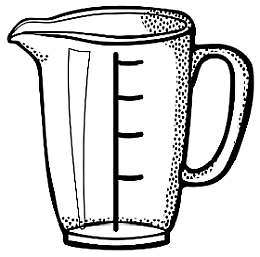# Ounces to Cups

Instructions: Use this calculator to convert ounces to cups, showing all the steps of the process. Please type the number of ounces you would like to convert in the form below:Type the number of ounces:

## Using this Ounces to Cups Conversion Tool

In the same way we did a conversion from cups to ounces, we can do the reverse process. Given a number of ounces, you may be interested in finding how many cups those ounces correspond to.

The process of converting volume units is fairly natural, considering that different countries may use radically different measures to quantify volumes and liquid content. The two main systems are the metric and the imperial.

Conversions are simple but typically, calculators and conversion tools are necessary, because it is not always easy to remember all the units and equivalences between the different systems.## What is 1 cup in Oz?

This the basis of all the analysis, based upon which we will find a general conversion formula. The basic equivalence is that 1 cup corresponds to 8 ounces, which can be used as the cornerstone for the general conversion process.

## How do you convert ounces to cups

Really, there is no mathematical trouble with making unit conversions and equivalences, but there as simply too many of them to remember them all .

## What are the steps for the conversion of ounces into cups

1. The first task is to observe that 1 cup corresponds with 8 fluid ounces. This is where we start from
2. Now, assume you have given O ounces. You want to know many cups C correspond to those O fluid ounces O
3. Now ,we establish a proportion: $$\displaystyle \frac{1}{8} = \frac{C}{O}$$
4. Solving the proportion shown above for C, we get the following conversion formula from ounces to cups: $$C = \displaystyle \frac{O}{8}$$

In this case and whenever we refer to the above formula, please remember that C refer to standard U.S. cups.

## Why would you want to convert ounces to cups

Different localities and in different contexts you will like use ounces, but cups is not an uncommon unit, so it may come to a situation where you will need to know the equivalence between cups and oz.

The metric system simplifies things a bit, because there is one main unit for volume, which is the liter, and the rest are derivatives of it, like the milliliter, etc.### Examples: Converting ounces to cups

Convert 10 ounces to cups

Solution: We need to identify the amount of ounces we want to convert, in this case we are given O = 10 ounces. The conversion formula is:

$C = \displaystyle \frac{O}{8}$

Therefore, now we need to plug in O = 10 ounces in the above formula:

$C = \displaystyle \frac{O}{8} = \displaystyle \frac{10}{8} = 1.25 \text{ cups}$

### Examples: Another ounces to cups conversion

Convert 2 cups to oz

Solution: The formula we have is:

$C = \displaystyle \frac{O}{8}$

But in this case we have C given, so we have

$C = \displaystyle \frac{O}{8} \Rightarrow 2 = \displaystyle \frac{O}{8}$ $\Rightarrow 2 \times 8 = O$ $\Rightarrow O = 16 \text {oz}$

## Other useful volume conversions

Computing from ounces to cups is a very common conversion. Perhaps a less common one but equally useful is the conversion cups to ounces, which is the reverse process to what we do in this calculator.

Ultimately, you may need lots of conversion tools, as you may need to deal with a whole array of units, especially if you mix the metric and imperial system.

Likely you will find useful converting from ounces to liters, and vice versa, from liters to ounces, which will make it possible to convert between metric and imperial.

Less commonly used conversion tools, but equally practical ones will deal with cups to quarts and quarts to gallons among many others.